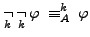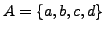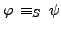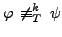# Algebraic properties of hyper-digital logic

online since July 2007

## Abstract

Having defined the syntax and semantics of hyper-digital logic (see ), this paper investigates the basic properties of the quasi-order relation on hyper-propositional formulas of a given carrier set A and degree k.

One set of properties immediately derives from the fact that these formula algebras are quasi-boolean algebras. We for example have

• the idempotency of conjunctions:• the associativity of disjunctions:• the law of double negation:Another set of properties involves the "box" and "diamond" operators and many results are similar to axioms and theorems of modal logic, such as
• the duality of box and diamond:But not all phenomena fit into the perspective of traditional logical systems:
• If sayis the carrier set then• Let S and T be two carrier sets, S a subset of T. In traditional propositional logic, we can always increase the carrier set at any time in the sense thatalways implies. But this is no longer true in hyper-propositional logic, where for each degree k there are two formulas withand.
From a deeper point of understanding of course, these "surprising" phenomena turn into perfectly normal behaviour.

## The text

The full text is currently under construction.